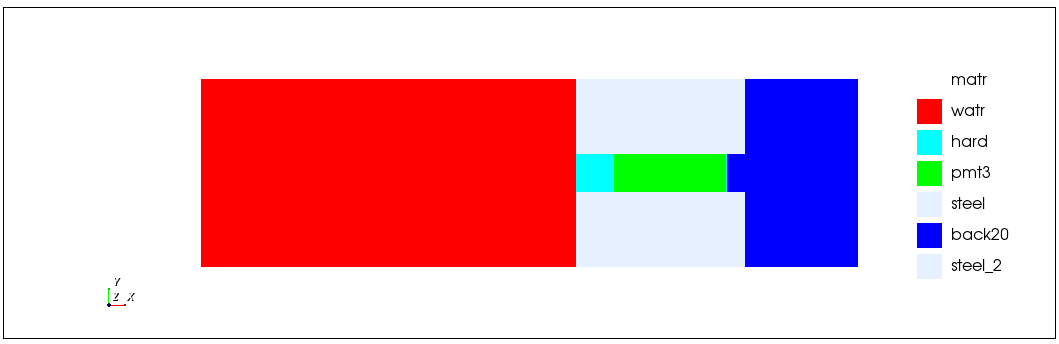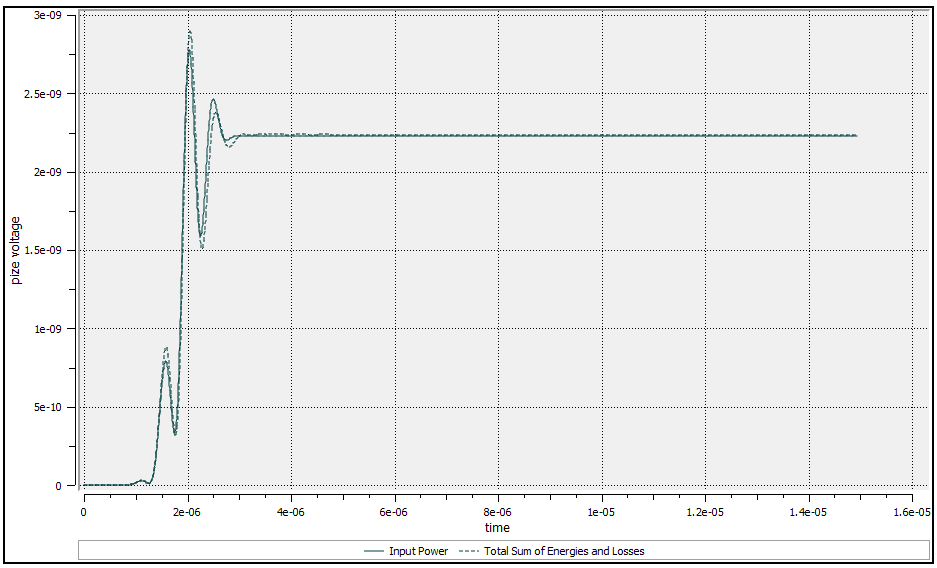# Calculating Energy Dissipation with CALC LOSS

## How to use Calc loss?

The calc loss command computes accumulated energy dissipation per unit volume.

Command can be issued before the PRCS step or at any time during the calculation.

Note: This command is currently implemented only for VDMP, SDMP, MDMP, RDMP and NWTN damping models. Implemented for small deformation 2D axisymmetric, 2D plane strain and 3D processors.

```c Calculations
calc
/* Request required arrays
strs
strn
loss inc
pres

/* Dissipated energy: Use avrg command to sum loss in array
avrg avloss loss volume regn \$i1 \$i2 \$j1 \$j2

/* Lateral energy: Energy lost through side
bwork brig \$i2 \$i2 \$j1 \$j2

end
```

Options: The calc loss command can be followed by any of ON, OFF or INC options:

• ON = compute energy loss during following time execution commands
• OFF = do not compute energy loss during following time execution commands
• INC = store the strain components needed for computing loss due to stiffness damping

Default = ON.

This command integrates the power dissipation per unit volume from the time an ON command is issued until an OFF command is encountered. When an ON command is encountered, the accumulated value is reset to zero.

The dissipated energy per unit volume values are stored in the LOSS (i,j,k) array. Where the ijk-indices are elemental indicies.

The strain components needed for computing loss due to stiffness damping are stored in arrays LSSHR(i,j,k) - shear damping coefficient, LSBLK(i,j,k) - bulk damping coefficient, LSD11(i,j,k), LSD22(i,j,k), LSD33(i,j,k), LSD12(i,j,k), LSD13(i,j,k), LSD23(i,j,k) - strain increments, LSTS(i,j,k) - timestep. Outputting in this format is useful for computing loss in the frequency domain. This is currently stored only for loss due to stiffness damping (SDMP).

## Example ModelThe following ultrasonic sensor example model calculates:

• Input electrical power via integration of Voltage * Current
• Dissipated energy via CALC LOSS
• Lateral leakage via CALC BWORKWe can verify that the total input energy is equal to the calculated energies and losses.

Result:

************************************************************************

Total sum of energies calculated 0.2900360E-08 Joules

Energy lost through heat 0.5148639E-09 Joules

Energy lost through sides 0.1718449E-08 Joules

Percentage lost as heat 17.75172 %

Percentage lost to sides 59.24951 %

************************************************************************

Post-process review script:

```/* Read history file

/* Time Histories Saved into the flxhst file
/* Loss through model boundaries
c    f1 3:'bwright'
c    f1 4:'bwleft'
c    f1 5:'bwbottom'
c    f1 6:'bwtop'

/* Loss through model boundaries
c    f1 7:'voltage'
c    f1 8:'charge'
c    f1 9:'current'

/* Calculation of 4 types of Energies
c    f1 13:'kinenrg'
c    f1 14:'elasenrg'
c    f1 15:'dielenrg'
c    f1 16:'coupenrg'

/* Average loss on all the model
c   20:'aloss'

/* Area scaling
symb ascal = 1.0

c Calculate total energy delivered
make
file f2
curv { f1 7 } * { f1 9 }
curv intg { f2 1 } /* Integration of UI to obtain input power
end

make

file f3
/* Calculate total energy from energies, loss and boundary losses
curv \$ascal * ( { f1 14 } + { f1 15 } + { f1 16 } + { f1 13 } + { f1 20 } + { f1 3 } + { f1 4 } + { f1 5 } + { f1 6 } )
/* Calculate Loss through sides only
curv \$ascal * ( { f1 3 } + { f1 4 } + { f1 5 } + { f1 6 } )
/* Calculate sum of 4 energies (kinenrg, elasenrg, dielenrg, coupenrg)
curv \$ascal * ( { f1 14 } + { f1 15 } + { f1 16 } + { f1 13 } )

end

grph
nvew 1
pset clab 1 'Input Power'
pset clab 2 'Total Sum of Energies and Losses'
plot f2 2 f3 1
end

/* Calculate efficiency
symb #get { * entot } curvmax f3 1
symb #get { * enside } curvmax f3 2
symb #get { * enloss } curvmax f1 20

symb ensum = \$entot - \$enside - \$enloss

symb pcloss = 100. * \$enloss / \$entot
symb pcside = 100. * \$enside / \$entot
symb econ = 100. * \$ensum / \$entot

/* Display results
symb #msg 10
************************************************************************
Total sum of energies calculated \$entot Joules
Energy lost through heat \$enloss Joules
Energy lost through sides \$enside Joules

Percentage lost as heat \$pcloss %
Percentage lost to sides \$pcside %
************************************************************************

term
```